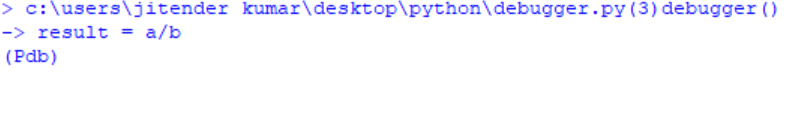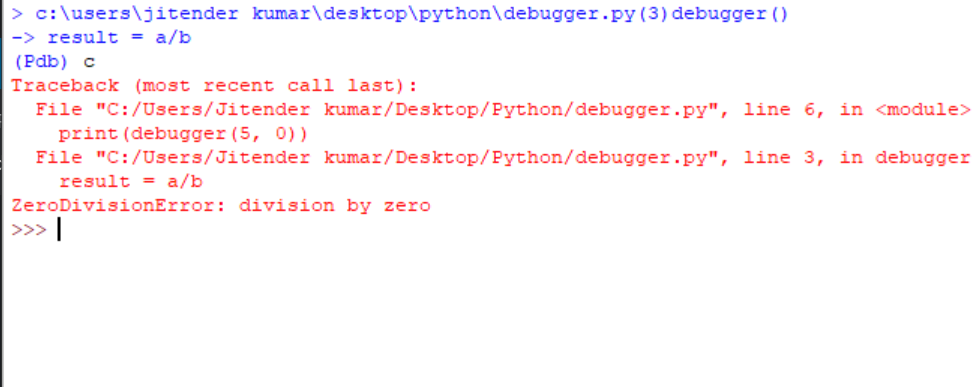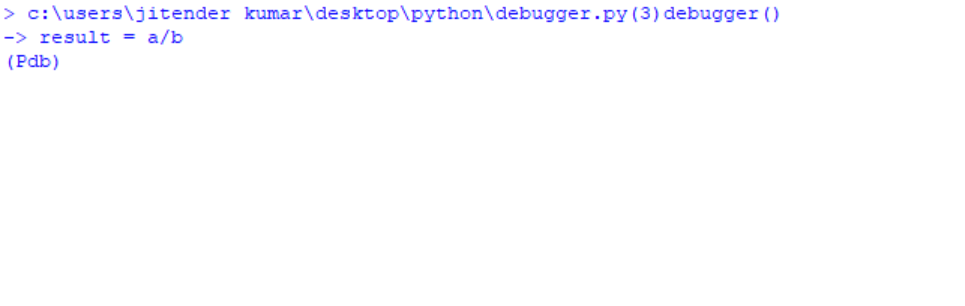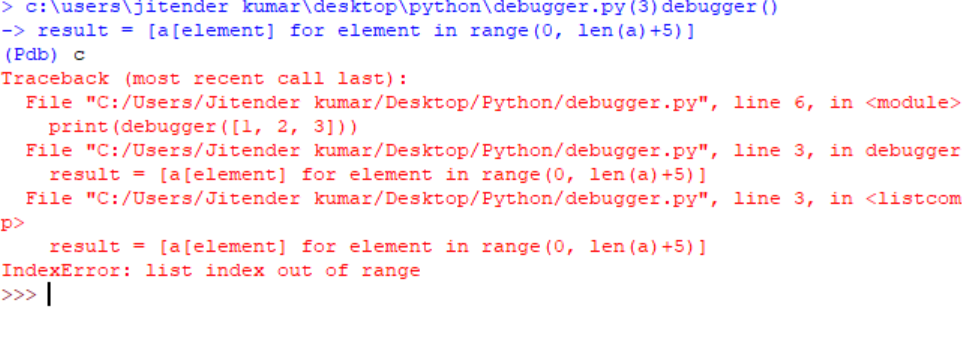# Debugging Python code using breakpoint() and pdb

• Difficulty Level : Medium
• Last Updated : 23 Feb, 2019

While developing an application or exploring some features of a language, one might need to debug the code anytime. Therefore, having an idea of debugging the code is quite necessary. Let’s see some basics of debugging using built-in function `breakpoint()` and `pdb module`.

We know that debugger plays an important role when we want to find a bug in a particular line of code. Here, Python comes with the latest built-in function breakpoint which do the same thing as `pdb.set_trace()` in Python 3.6 and below versions.

Debugger finds the bug in the code line by line where we add the breakpoint, if a bug is found then program stops temporarily then you can remove the error and start to execute the code again.

Syntax:

```1) breakpoint()           # in Python 3.7

2) import pdb; pdb.set_trace()   # in Python 3.6 and below
```

Method #1 : Using breakpoint() function
In this method, we simply introduce the breakpoint where you have doubt or somewhere you want to check for bugs or errors.

 `def` `debugger(a, b):``    ``breakpoint()``    ``result ``=` `a ``/` `b``    ``return` `result`` ` `print``(debugger(``5``, ``0``))`

Output :In order to run the debugger just type c and press enter.Commands for debugging :

```c -> continue execution
q -> quit the debugger/execution
n -> step to next line within the same function
s -> step to next line in this function or a called function
```

Method #2 : Using pdb module
As the same suggests, PDB means Python debugger. To use the PDB in the program we have to use one of its method named `set_trace()`. Although this will result the same but this the another way to introduce the debugger in python version 3.6 and below.

 `def` `debugger(a, b):``    ``import` `pdb; pdb.set_trace()``    ``result ``=` `a ``/` `b``    ``return` `result`` ` `print``(debugger(``5``, ``0``))`

Output :In order to run the debugger just type c and press enter.Example :

 `def` `debugger(a):``    ``import` `pdb; pdb.set_trace()``    ``result ``=` `[a[element] ``for` `element ``in` `range``(``0``, ``len``(a)``+``5``)]``    ``return` `result`` ` `print``(debugger([``1``, ``2``, ``3``]))`

Output :My Personal Notes arrow_drop_up